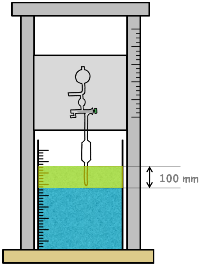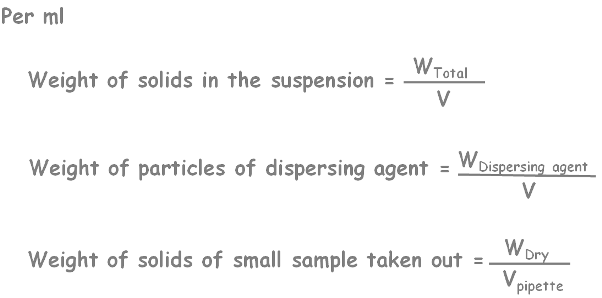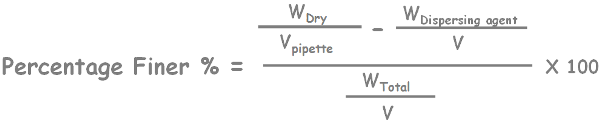Sedimentation Analysis - Pipette Method

Pipette method is one of the methods of taking observation of liquid soil suspension for the purpose of sedimentation analysis of soils containing fine particles.

Theory of sedimentation, its limitations and the method of preparation of soil suspension have already been discussed, read them here.

For the preparation of soil suspension we took some 50 gm of oven dried soil sample which weighed as Wtotal.

We mix 100 ml of dispersing agent to our taken sample.

Dispersing agent is prepared by mixing two components to 1 litre of distilled water.

The weight of dispersing agent’s particles is Wdispersing agent.

And finally distilled water was added to make the solution 1000 ml.

Lets say this total volume as capital V.For pipette method we need only 500 ml of soil suspension.

We require this kind of arrangement to take out a small portion of suspension from a specified depth time to time. Sedimentation jar is placed below this pipette.

First we determine the volume of the pipette. For that we use distilled water. We immerse the nozzle of the pipette into this water and using stop cock arrangement we suck up the water in the pipette. Then we discharge this water into measuring jar and volume of the pipette can be known. Lets say this volume as Vpipette.

Now for the sampling, first sedimentation jar containing the prepared soil suspension is placed in constant temperature of 27°C for about an hour. The jar is then taken out and a rubber bung is placed at its top to close its mouth. Then tube is inverted a few times so that suspension becomes homogeneous. Bung is removed and jar is placed over the constant temperature bath. The pipette arrangement is fixed over the jar and a stop watch is started to record the time of settlement of particles.

Particles in the suspension start settling down. According to the equation…Large particles settles with greater speed than small particles do. So at a certain depth we will find different concentrations of different particles at different times. But in the beginning of the sedimentation because of suspension being homogenous, particles of all sizes present at all the depths.When 2 minutes elapsed then we take our first sample. Pipette is gradually lowered to the depth of 100 mm into the suspension about 20 seconds before the sample is to be taken.

By our established formula we can determine in time 2 mins, that is 120 seconds, D sized soil particle would have traveled to the 100 mm distance. So in and above this layer we will find all the particles of size smaller than D.We will be taking all our samples from this depth.

The sample is sucked into the pipette using stop cock and pipette is taken out.

The taken sample is then transferred to the weighing bottle. If any particle remains adhered to the tube, the distilled water is used to transfer it into the bottle. Then the sample is dried in oven at 105°C to 110°C for 24 hours.

After drying weight of the sample is taken and say it as WDry.

Now here we have

total weight of solids in the suspension.

Weight of particles of dispersing agent.

and weight of solids of small sample taken after 2 mins W(Dry), which has only particles smaller than a particular diameter.

But all of weight of solids W(Total) contained in the volume capital V which we took as 1000 ml.

And weight of particles of dispersing agent W(Dispersing Agent) is also contained in the volume V.

But weight of solids of extracted sample W(Dry) are in the volume of pipette V(pipette) which is only 10 ml.

So lets represent each in per ml weight so that we can compare them with each other.As we know percentage finer is weight of sample with particles of size smaller than D divided by weight of the total soil sample with all the particles present in suspension.

As our extracted dried sample contains all the particles of size smaller than D and total sample has particles of all sized in it.

So in the percentage finer equation lets substitute per ml weight of extracted sample which contains all the particles smaller than size D.

And in the denominator substitute per ml weight of all the particles of all sizes.

Also notice that this extracted sample also has some particles of dispersing agent, so lets remove their concentration in the equation.This is how we calculate the percentage finer with the pipette method.

In similar fashion more samples are taken after 4, 8, 15 and 30 mins and 1, 2, 4, 8 and 24 hours and percentage finer for different sized particles can be known.

This method’s merit is that it is very accurate.

But demerits are:

Apparatus is quite delicate and expensive.

It also requires very sensitive weighing balance for smaller extracted samples from the suspension.

Also The procedure is very long.

For quick particle size analysis of fine particles hydrometer method is more suitable.

Tags : Sedimentation, soil test

Published on :2019-09-06